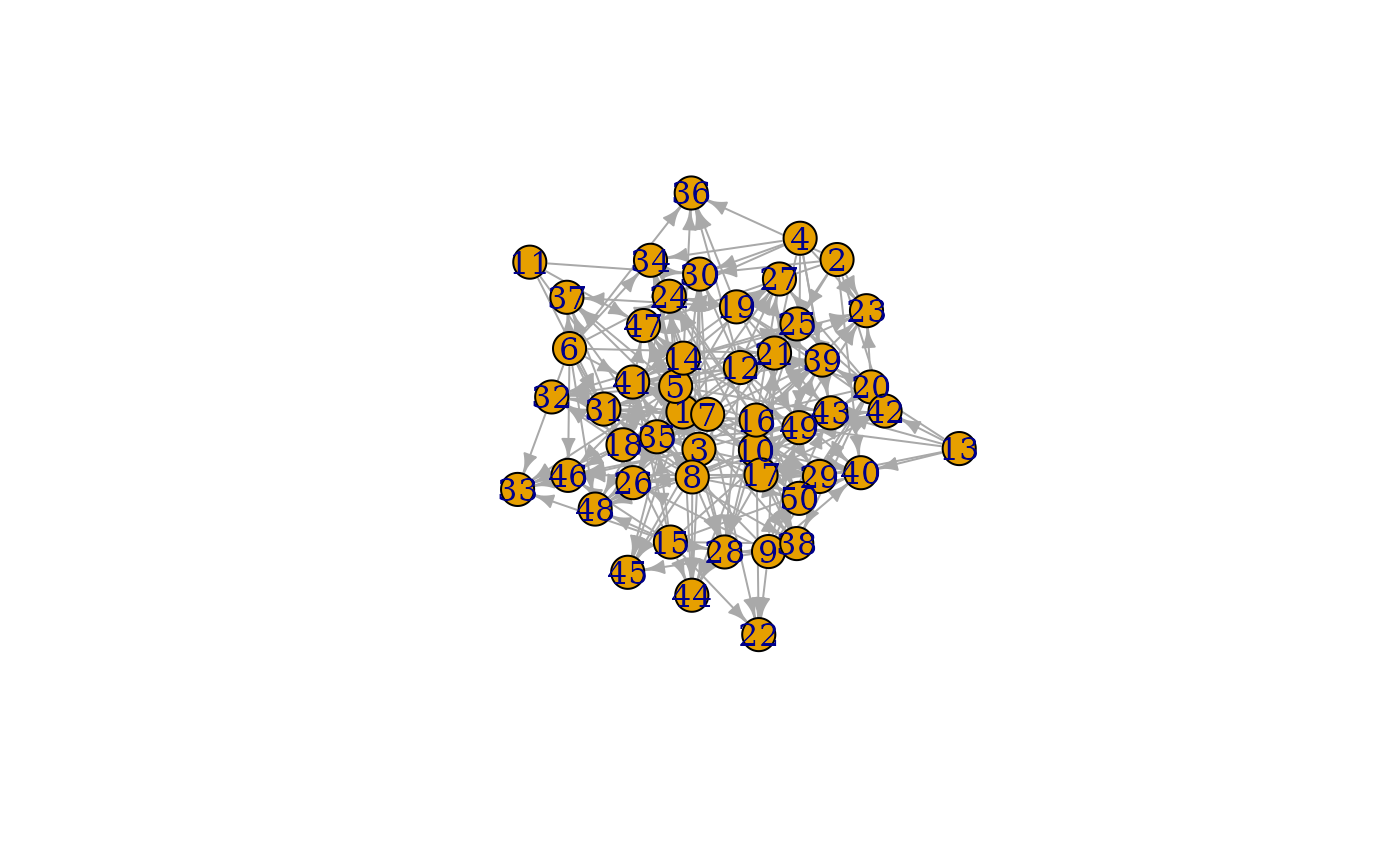This set of games are build around different types of nodes and simulating their interaction. The nature of their algorithm is described in detail at the linked igraph documentation.

play_preference(
n,
n_types,
p_type = rep(1, n_types),
p_pref = matrix(1, n_types, n_types),
fixed = FALSE,
directed = TRUE,
loops = FALSE
)

play_preference_asym(
n,
n_types,
p_type = matrix(1, n_types, n_types),
p_pref = matrix(1, n_types, n_types),
loops = FALSE
)

play_bipartite(n1, n2, p, m, directed = TRUE, mode = "out")

play_traits(
n,
n_types,
growth = 1,
p_type = rep(1, n_types),
p_pref = matrix(1, n_types, n_types),
callaway = TRUE,
directed = TRUE
)

play_citation_type(
n,
growth,
types = rep(0, n),
p_pref = rep(1, length(unique(types))),
directed = TRUE
)

## Arguments

n, n1, n2 The number of nodes in the graph. For bipartite graphs n1 and n2 specifies the number of nodes of each type. The number of different node types in the graph The probability that a node will be the given type. Either a vector or a matrix, depending on the game The probability that an edge will be made to a type. Either a vector or a matrix, depending on the game Should n_types be understood as a fixed number of nodes for each type rather than as a probability Should the resulting graph be directed Are loop edges allowed The probabilty of an edge occuring The number of edges in the graph The flow direction of edges The number of edges added at each iteration Use the callaway version of the trait based game The type of each node in the graph, enumerated from 0

## Value

A tbl_graph object

## Functions

• play_preference: Create graphs by linking nodes of different types based on a defined probability. See igraph::sample_pref()

• play_preference_asym: Create graphs by linking nodes of different types based on an asymmetric probability. See igraph::sample_asym_pref()

• play_bipartite: Create bipartite graphs of fixed size and edge count or probability. See igraph::sample_bipartite()

• play_traits: Create graphs by evolving a graph with type based edge probabilities. See igraph::sample_traits() and igraph::sample_traits_callaway()

• play_citation_type: Create citation graphs by evolving with type based linking probability. See igraph::sample_cit_types() and igraph::sample_cit_cit_types()

Other graph games: component_games, evolution_games, sampling_games
plot(play_bipartite(20, 30, 0.4))